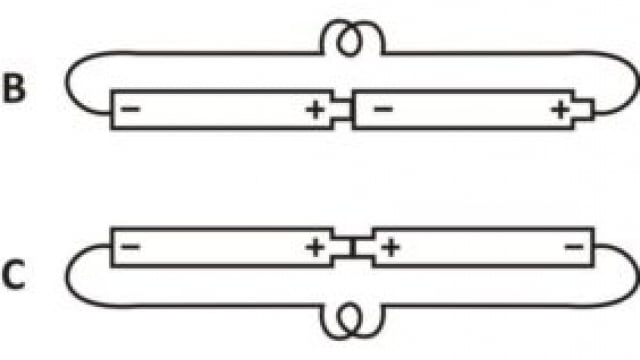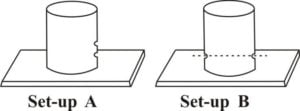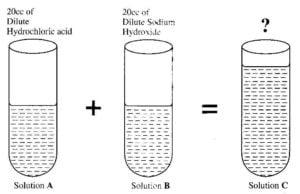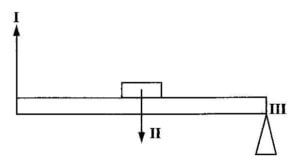Home / Science / BECE Past Questions & Answers – 2005 (SCIENCE)

# BECE Past Questions & Answers – 2005 (SCIENCE)April2005

SECTION B
1 hour15 minutes
ESSAY [75marks]

This section is in two parts, I and II

AnswerQuestion1ofPartIand any other three questions in PartII.

Credit will be given for clarity of expression and orderly presentation of material

PART I
[30 marks]

1. (a)In an experiment, a pupil took two empty Milo tins and made holes in their sides as shown in the diagram above.The pupil then filled the Milo tins with water.

(i) Draw and label the diagrams to show what the pupilwillobservein set-up A and set-up B

(ii) Explain the observations in set-up A and set-up B
(iii) What is the aim of set-up A? (iv) What is the aim of set-up B?

(b) In an experiment, equal volumes of dilute hydrochloric acid (solution A) and dilute sodium hydroxide (solution B) are mixed together to form solution C.(i) What is the volume of solution C?

(ii) Red litmus paper and blue litmus paper are dipped in turns into solutions A,Band C.

State the observations you will make in all six cases

(iii) Give the name of the reaction that took place between solution A and solution B

(iv) Identify solution C

(v) State what will happen when solution C is heated.

(c) The table below gives the steps that were followed in an experiment to test for starch in a green leaf freshly taken from a tree.

Stages in the test for starch
I. Leaf is put into boiling water for1 minute
II. Leaf is dipped in alcohol warmed in a hot water bath
III. Leaf is washed in cold water
IV. Leaf is dipped into iodine solution

(i) State the reason for carrying out each of the activities in stages I, II and III. (ii) What happens when the leaf is dipped in iodine solution?

(iii) Give the colour changes of the leaf from the beginning of the experiment to the end of the experiment.
(iv) Why is the alcohol warmed indirectly in a water bath?
(v) Explain what will be observed if the test is carried out on a leaf taken from a plant kept in a dark room for1 day [10 marks]

PART II
[45 marks]

Answer three questions from this part

2.

(a)

(i) (ii)

Define respiration.
Give two differences between photosynthesis and respiration.

(b)
(i) (ii)
List the chemicals used in the preparation of carbon dioxide in the laboratory. How would you test for carbon dioxide in the laboratory?

(c)
(i) (ii)
State four effects of a force on a body.
A horizontal force of 250N is applied to pull a piece of wood lying on a smooth surface through a distance of 20m. Calculate the work done.

3. (a) (i) (ii) (iii) Give two differences between a vein and an artery. State two functions of red blood cells.
Mention two diseases associated with the circulatory system.

(b)
(i)
Classify the following processes into a physical change and a chemical change

Cooking of food; Melting of candle; Melting of ice; Rusting of iron;
Dissolution of sugar in water; Burning of wood into ash.

(ii)
Explain the basis of the classification in (b) (i)

(c)
(i) (ii)
State the laws of reflection
Draw a ray diagram of light incident at an angle of 40° on the surface of a plane mirror.

4. (a) (i) Explain vegetative reproduction
(ii) State two differences between sexual reproduction and vegetative reproduction
(iii) Give one advantage of vegetative reproduction

(b) (i) Name suitable solvents for the following substances: Oil paint, fat, common salt, plant pigments.
(ii) What is the name given to a substance dissolved by a solvent?

(c)The diagram above represents a simple machine

(i) What class of simple machine does it represent? (ii) Name the parts labeled I,II, and III.
(iii) Give two examples of machines used in everyday life which work on the same principle as the system illustrated above.

5. (a) (i) (ii) Give two differences between a parasite and a vector.
Write down the names of three endo-parasites and their hosts.

(b) An atom has eight protons and nine neutrons.
(i) Draw the atomic structure of the atom.
(ii) If the atom gains two extra electrons what will be the charge of the ion formed?
(Show working)

(c)
(i)
Three insoluble liquids X, Y and Z are shaken together in a bottle and allowed to settle.
The densities of the liquids are as follows:
X =1.5g/cm3;Y =3.0 g/cm3and Z=1.0g/cm3.

Draw a sketch to show the various layers into which the liquids settle.